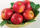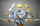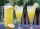# Fraction calculator

The calculator performs basic and advanced operations with fractions, expressions with fractions combined with integers, decimals, and mixed numbers. It also shows detailed step-by-step information about the fraction calculation procedure. Solve problems with two, three, or more fractions and numbers in one expression.

## Result:

### (8/9 - 1/4)-(1/2 + 5/6) = -25/36 ≅ -0.6944444

Spelled result in words is minus twenty-five thirty-sixths.

### How do you solve fractions step by step?

1. Subtract: 8/9 - 1/4 = 8 · 4/9 · 4 - 1 · 9/4 · 9 = 32/36 - 9/36 = 32 - 9/36 = 23/36
For adding, subtracting, and comparing fractions, it is suitable to adjust both fractions to a common (equal, identical) denominator. The common denominator you can calculate as the least common multiple of both denominators - LCM(9, 4) = 36. In practice, it is enough to find the common denominator (not necessarily the lowest) by multiplying the denominators: 9 × 4 = 36. In the following intermediate step, the fraction result cannot be further simplified by canceling.
In other words - eight ninths minus one quarter = twenty-three thirty-sixths.
2. Add: 1/2 + 5/6 = 1 · 3/2 · 3 + 5/6 = 3/6 + 5/6 = 3 + 5/6 = 8/6 = 2 · 4/2 · 3 = 4/3
For adding, subtracting, and comparing fractions, it is suitable to adjust both fractions to a common (equal, identical) denominator. The common denominator you can calculate as the least common multiple of both denominators - LCM(2, 6) = 6. In practice, it is enough to find the common denominator (not necessarily the lowest) by multiplying the denominators: 2 × 6 = 12. In the following intermediate step, cancel by a common factor of 2 gives 4/3.
In other words - one half plus five sixths = four thirds.
3. Subtract: the result of step No. 1 - the result of step No. 2 = 23/36 - 4/3 = 23/36 - 4 · 12/3 · 12 = 23/36 - 48/36 = 23 - 48/36 = -25/36
For adding, subtracting, and comparing fractions, it is suitable to adjust both fractions to a common (equal, identical) denominator. The common denominator you can calculate as the least common multiple of both denominators - LCM(36, 3) = 36. In practice, it is enough to find the common denominator (not necessarily the lowest) by multiplying the denominators: 36 × 3 = 108. In the following intermediate step, the fraction result cannot be further simplified by canceling.
In other words - twenty-three thirty-sixths minus four thirds = minus twenty-five thirty-sixths.

#### Rules for expressions with fractions:

Fractions - use the slash “/” between the numerator and denominator, i.e., for five-hundredths, enter 5/100. If you are using mixed numbers, be sure to leave a single space between the whole and fraction part.
The slash separates the numerator (number above a fraction line) and denominator (number below).

Mixed numerals (mixed fractions or mixed numbers) write as non-zero integer separated by one space and fraction i.e., 1 2/3 (having the same sign). An example of a negative mixed fraction: -5 1/2.
Because slash is both signs for fraction line and division, we recommended use colon (:) as the operator of division fractions i.e., 1/2 : 3.

Decimals (decimal numbers) enter with a decimal point . and they are automatically converted to fractions - i.e. 1.45.

The colon : and slash / is the symbol of division. Can be used to divide mixed numbers 1 2/3 : 4 3/8 or can be used for write complex fractions i.e. 1/2 : 1/3.
An asterisk * or × is the symbol for multiplication.
Plus + is addition, minus sign - is subtraction and ()[] is mathematical parentheses.
The exponentiation/power symbol is ^ - for example: (7/8-4/5)^2 = (7/8-4/5)2

#### Examples:

subtracting fractions: 2/3 - 1/2
multiplying fractions: 7/8 * 3/9
dividing Fractions: 1/2 : 3/4
exponentiation of fraction: 3/5^3
fractional exponents: 16 ^ 1/2
adding fractions and mixed numbers: 8/5 + 6 2/7
dividing integer and fraction: 5 ÷ 1/2
complex fractions: 5/8 : 2 2/3
decimal to fraction: 0.625
Fraction to Decimal: 1/4
Fraction to Percent: 1/8 %
comparing fractions: 1/4 2/3
multiplying a fraction by a whole number: 6 * 3/4
square root of a fraction: sqrt(1/16)
reducing or simplifying the fraction (simplification) - dividing the numerator and denominator of a fraction by the same non-zero number - equivalent fraction: 4/22
expression with brackets: 1/3 * (1/2 - 3 3/8)
compound fraction: 3/4 of 5/7
fractions multiple: 2/3 of 3/5
divide to find the quotient: 3/5 ÷ 2/3

The calculator follows well-known rules for order of operations. The most common mnemonics for remembering this order of operations are:
PEMDAS - Parentheses, Exponents, Multiplication, Division, Addition, Subtraction.
BEDMAS - Brackets, Exponents, Division, Multiplication, Addition, Subtraction
BODMAS - Brackets, Of or Order, Division, Multiplication, Addition, Subtraction.
GEMDAS - Grouping Symbols - brackets (){}, Exponents, Multiplication, Division, Addition, Subtraction.
Be careful, always do multiplication and division before addition and subtraction. Some operators (+ and -) and (* and /) has the same priority and then must evaluate from left to right.

## Fractions in word problems:

• Pie division5/8 of a pie divide 6 pieces. Each friend got 1/6.  What fraction  of the whole pie does each person receive?
• A quotientWhat is the quotient of 3/10 divided by 2/4 as a fraction?Sadie picked up fallen apples from an apple tree in her yard. She picked up 14 green ones, 16 that were changing from green to red, and 18 that were red. What fraction in simplest form names the green apples?
• Frac equation v23.80 is two fifths of a quarter sum of money. How much money is there?
• EqnSolve equation with fractions: 2x/3-50=40+x/4Max is working out 2/3+7/9. He says the answer is 9/12. What mistake have Max made?
• Write 2Write 791 thousandths as fraction in expanded form.
• Divide 13Divide. Simplify your answer and write as an improper fraction or whole number. 14÷8/3
• Ratio 11Simplify this ratio 10 : 1/4Six friends are sharing 8 cups of lemonade. If they share the lemonade equally, how many cups will each friend get?Andre, Thomas, and Ivan split 88 postage stamps in a 2:5:4 ratio. How much did Thomas get?Sum of two fractions is 4 3/7. If one of the fractions is 2 1/5 find the other one .Solve the following equation: 2x- (8x + 1) - (x + 2) / 5 = 9